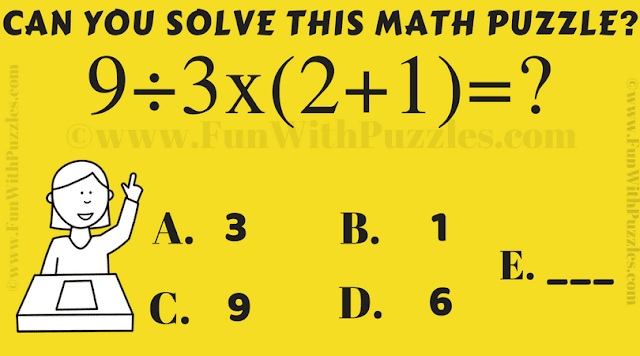Step into the world of numbers and quick thinking with our engaging Quick Calculate Math Puzzle, specially designed for school kids. This puzzle is more than just a mathematical challenge—it's a test of your fundamental math skills and your ability to swiftly dissect and solve problems.

Within this puzzle lies the opportunity to showcase your grasp of the fundamentals, utilizing the principles of PEMDAS or BODMAS—order of operations in mathematics. As you navigate through the equation, remember that each step holds significance and precision is key.Can you solve this Math Puzzle quickly?

But this isn't just about solving math problems; it's also a race against the clock. As you embrace the challenge, the timer ticks away, urging you to find the solution with speed and accuracy. How swiftly can you solve this puzzle? Your time is a testament to your math proficiency and your quick thinking.

The beauty of this math puzzle is its blend of learning and excitement. It's a reminder that math isn't just about equations; it's a tool that sharpens your analytical abilities and nurtures your problem-solving agility.

Embark on this mathematical journey, and witness the joy of unlocking the puzzle's solution in record time. As you unravel the intricacies of numbers and operations, you're not just solving a puzzle—you're conquering new heights of mathematical mastery.

The answer to this "Quick Calculate Mathematical Puzzle", can be viewed by clicking on the button. Please do give your best try before looking at the answer.

To solve this quick calculate Mathematical puzzle correctly on your first attempt, you require good Mathematical skills. Check out the below-listed puzzles that will test your mathematical knowledge, Logical Reasoning, and Observational power.

## List of Maths, Logic, and Visual Puzzles

### Conceptis Puzzles

These are similar puzzles to Calcudoku which can be played online. These puzzles are by Conceptis Puzzles.

### Brain Teasers & Riddles IQ Test for Teens & Adults with Answers

This is a mix of Mathematical puzzles and Logical puzzles which will test your IQ.

These are very challenging Brain Teasers in which your challenge is to find the missing number in the Circle.

### Interesting Brain Teasers to Challenge Your Mind

It only contains Mathematical or Logical Puzzles but also Visual Brain Teasers in which your challenge is finding the hidden animals in the picture puzzle image.